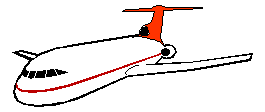PRINT THIS ANSWER SHEET TO FILL OUT AND TURN IN.

NAME_________________________________ CLASS____________________ DATE____________

Size Effects Worksheet1. What happens to lift when the surface area of an airplane's wings is increased?
_______________________________

2. Open the Web page (http://en.wikipedia.org/wiki/Boeing_747), or a search engine, to find information about the Boeing 747. What is its wing area?_________________________

3. Refer to the above question. What is the Boeing 747's takeoff weight?__________________

4. How many passengers can the Boeing 747 carry? ______________________

5. What is the ratio of the Boeing 747's takeoff weight to its wing area? __________________

6. Now use the Web page (http://en.wikipedia.org/wiki/Boeing_777), or a search engine, to find information about the Boeing 777. What is its wing area?
___________

7. What is the takeoff weight of the Boeing 777? ___________________

8. How many passengers can the Boeing 777 carry? ___________________

9. What is the ratio of the Boeing 777's takeoff weight to its wing area? _______________

10. Look up the DC-8 at (http://en.wikipedia.org/wiki/DC-8), or use a search engine. What is its wing area? __________________

11. What is the DC-8's takeoff weight? ______________________

12. How many passengers can the DC-8 carry? _______________________

13. What is the ratio of the DC-8's takeoff weight to its wing area?___________________

14. Find the DC-10 at (http://en.wikipedia.org/wiki/DC-10), or use a search engine . What is its wing area? ____________________

15. What is the DC-10's takeoff weight? _________________________

16. How many passengers can the DC-10 carry? __________________________

17. What is the ratio of the DC-10's takeoff weight to its wing area? _______________

18. If both the Boeing 777 and the DC-10 weighed 130,000 kg, which airplane's wings would generate the most lift? ______________________

19. Which of the two planes in the previous question has a greater possible takeoff weight? ___________________

20. Which two of the four jet airplanes above have the closest takeoff weight to wing area ratios? ________________________

21. Explain the relationships among lift, takeoff weight, and wing area. You may want to read the slide called Forces on an Airplane. ___________________________________________

22. Do you see a relationship between wing span and passenger number? _________________

23. Where are slats located and how do they affect an airplane's lift?______________________

24. Where are the flaps located? ____________________